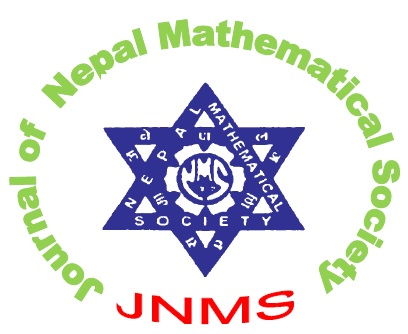Journal of Nepal Mathematical Society (JNMS)
Developing the Society Through Mathematical Research

ISSN: 2616-0153 (Print) ISSN: 2616-0161 (Online)Aim & Scope

The Journal of Nepal Mathematical Society (JNMS) is the official journal published by the Nepal Mathematical Society (NMS). It covers wide spectrum of the field of mathematics and its real-world applications.

This journal will be publishing the high-quality theoretical results, new mathematical models and algorithms, efficient computational studies in any field of pure and applied mathematics. The journal also emphasizes the original mathematical papers devoted to the mathematical sciences with mathematical treatment of questions arising in the real-life problems, like computer science/informatics, physics, chemistry, biology, medicine, engineering, management and all applied sciences. The article should have significant contributions on conceptual development of pure or applied mathematics or on their current developments and practices where the applicability can be explicitly materialized.

The journal JNMS publishes full-length original research articles, state-of-the arts surveys, high standard short notes in any field of mathematics. The pure and applied fields include, but is not limited to, algebra, mathematical analysis, complex analysis, functional analysis, topology, algebraic topology, geometry, algebraic geometry, differential geometry, differential equations, dynamical systems, continuous and discrete optimization, operations research, scheduling theory, graph theory, biomathematics, industrial mathematics, financial mathematics, mathematical modeling, computational mathematics, number theory, control theory, mathematical statistics, and any area of mathematical sciences.